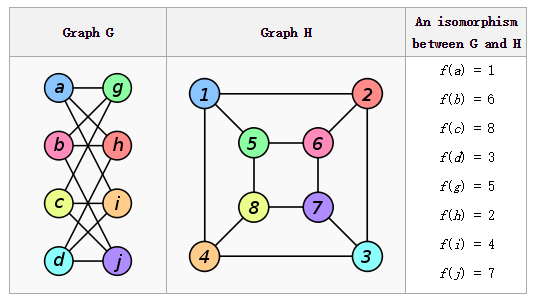# 有趣的交互式证明P有一只红袜子和黄袜子，她的一个色盲朋友V不相信P的袜子颜色不同，P如何才能让V相信这是真的呢？一个简单的办法如下：

1. P把两只袜子给V，V每只手拿了一只袜子
2. P转过身背对V
3. V抛一枚硬币，如果头面朝上，则保持两只袜子不动，否则交换左右手的袜子
4. P转过身，V问P是否交换过袜子
5. 如果P回答错误，则V不相信；否则，重复100次实验，如果P都回答正确，则V相信这两只袜子是不同颜色的

$k$$N\rightarrow N$的一个函数，我们称语言$L$属于$IP[k]$，如果存在一个$k(|x|)$多项式时间概率图灵机TM $V$，使得：

IP这个复杂性类就是所有IP[k]的并集。在IP中，P的能力是无穷的，但它不一定是诚实的；V能力较弱，只能进行多项式时间的计算。1. V：随机选一个$i\in \{1,2\}$，对$G_i$做一个随机的置换，得到新图$H$，则有$H\cong G_i$，将$H$发送给P
2. P：发送$j$给V
3. V：如果$i\neq j$，则拒绝；否则重复100次实验，都有$i==j$，则相信$G_1\ncong G_2$

P.S.

1. V：取模$p$的随机数$r(\mod p)$和随机数$b\in\{0,1\}$，如果$b=0$，发送$r^2(\mod p)$给P；否则发送$a\cdot r^2(\mod p)$给P
2. P：发送$b'$给V
3. V：如果$b'\neq b$，则拒绝；否则重复100次实验，都有$b'=b$，则相信$\in QNR$

## 2 thoughts on “有趣的交互式证明”

1.Sweetfish Li

为什么交互式证明正确的概率不是1 而是大于三分之二

2.nan